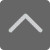## 爱智康资讯站

### 课程咨询: 4000-121-121

• 全国课程在线咨询
• 咨询热线：4000-121-121
• 登录手机官网

• 关注微信公众号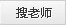# 【通俗数学】分数和无限循环小数为什么可以互相转化？

2020-02-19 17:08:20 　来源：西安爱智康 文章作者：西安爱智康

## 学而思・爱智康1对1/8人班 帮助孩子解决知识难点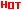*2470人已预约，首课体验不满意，可全额退费。

― ― 报名课程可获得 ― ―

0.9999.....＝1

2/9=0.2222222222.....

112/99=1+13/99=1.1313131313.....

325/999=0.325 325 325 325.....

5/3=15/9=1+6/9=1.6666666......

5/11=45/99=0.45454545......

10/37＝270/999=0.270270270......

5/7＝714285/999999

=0.714285 714285 714285......

7/15  → 70/15=14/3=4+2/3=4.666666.......

3/28   →    300/28=75/7＝10+5/7＝

10+714285/999999＝10.714285 714285 714285......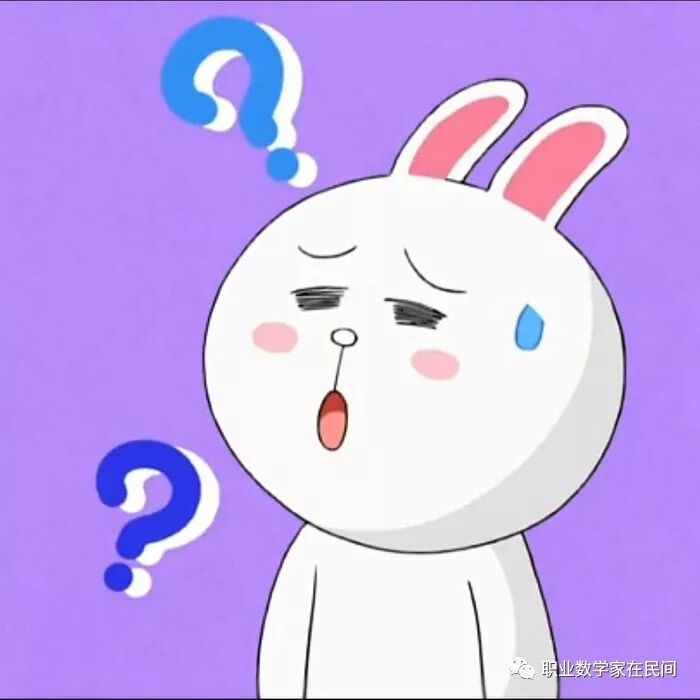7/15→70/15=14/3

3/28→300/28=75/7

5/7＝714285/999999

1001/999=1＋2/999=1.002002002......

(欧拉定理)设a是与n互素的正整数，则

aφ(n)－1被n整除

999999=106－1被7整除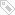标签：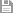保存　｜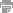打印　｜关闭